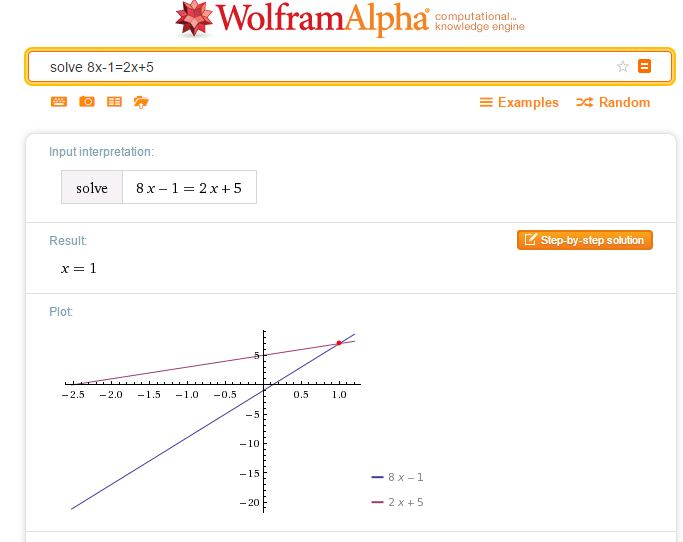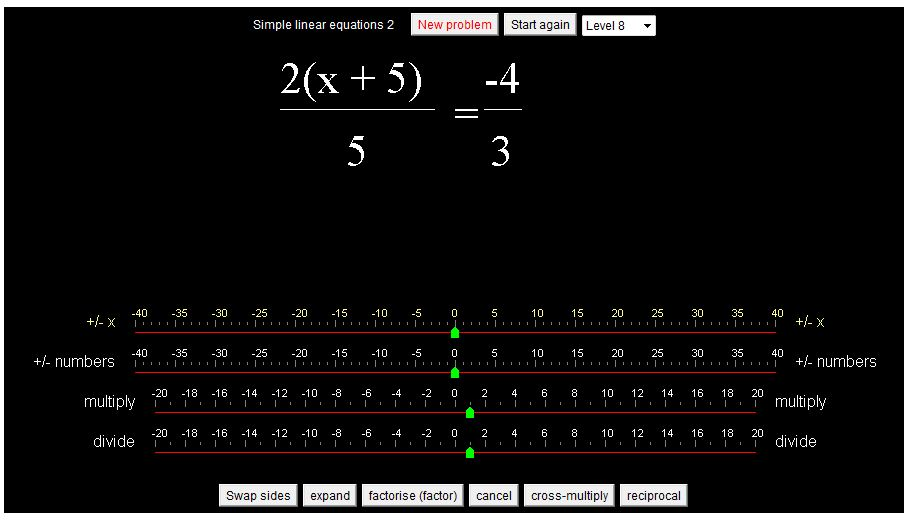# From Year 7 to Year 13…

Such a busy week…

But I have written a weekly blog post since January 2011 when I made a New Year resolution to write a blog post every week, a habit I don’t intend to stop, so this week I simply offer two slideshows I created for my students recently.

With Year 7 (age 11-12) we have been studying Algebra. When solving equations, I included for a class of high ability students, equations with the unknown on both sides. Having given them one homework where they were exploring various resources for practising solving equations, a sudent asked in a comment on our homework blog how to use Duncan Keith’s excellent linear equation calculator for practising this type of equation:

The slideshow below shows how to use the calculator to solve equations where the unknown is on both sides.

.
At the other end of the school, with Year 13 I had completed the various integration techniques required for our exam specification. Aware that students sometimes muddle differentiation and integration, I started the last lesson of the series with one of my ‘self-checks’ / mini tests to see what they could easily recall. I have stressed the importance of knowing the basics with this group. The questions I used are presented in the following slide show – a sort of KS5 mental Calculus test!

# Solving Equations

A collection of resources to use to demonstrate and practise solving equations.A simple way to check a solution to an equation of any type is to simply enter your equation as a WolframAlpha query. Note that WolframAlpha includes a graphical illustration; it is so important for students to understand how equations may be solved graphically; I always illustrate graphical solutions when we are working with Algebra to help students make these links. Desmos of course, is ideal here.The following resources allow practice in choosing the correct operations to reach a solution.

Centre for Innovation in Mathematics Teaching
CIMT
have tutorials on equations: Linear Equations 1, Linear Equations 2, and Linear Equations with Brackets in their Interactive ResourcesChoose the type of equation you require then the sequence of operations required to solve the equation.
Select Do it after each operation, for example -32 Do it were the keys selected to start the above problem.

The next sites work in a similar way to the subtangent resource.

Flashy MathsSolving Equations (select the swf file to play online)There are 4 levels to choose from: the level 1 equations are of the type ax = b,
level 2, x±c = d, level 3, ex±f = g and level 4, hx±i = hx±j = k.
Choose the series of operations you require to solve the equation selecting apply at each stage to see the result of your chosen operation.
Choose numbers to add and subtract integers and letters to add and subtract variables.

MathsNetEquation Buster
Playing at level 5 introduces equations with fractions.From the National Library of Virtual Manipulatives try Algebra Balance Scales and Algebra Balance Scales – Negatives.
Note that these manipulatives allow you to create a problem of your own (so long as it has an integer solution) as well as solving problems given.From WaldoMaths, try this applet on solving simple linear equations and this on equations requiring more complex manipulation.
Each applet has several levels.Unlike the other resources this allows the user to enter the equation resulting from the chosen operation.
The solution is checked at each stage.
As well as solving the given problems (20 problems) the user can select ‘Make an equation yourself’ to enter any equation then solve that equation.
(Unlike the NLVM resource above the x can be any real number).

The following video shows the keystrokes required to enter and solve an equation.